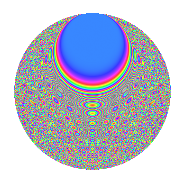# Properties

 Label 804.2.uLevel 804 Weight 2 Character orbit u Rep. character $$\chi_{804}(43,\cdot)$$ Character field $$\Q(\zeta_{22})$$ Dimension 680 Newforms 2 Sturm bound 272 Trace bound 3

# Related objects

## Defining parameters

 Level: $$N$$ = $$804 = 2^{2} \cdot 3 \cdot 67$$ Weight: $$k$$ = $$2$$ Character orbit: $$[\chi]$$ = 804.u (of order $$22$$ and degree $$10$$) Character conductor: $$\operatorname{cond}(\chi)$$ = $$268$$ Character field: $$\Q(\zeta_{22})$$ Newforms: $$2$$ Sturm bound: $$272$$ Trace bound: $$3$$ Distinguishing $$T_p$$: $$7$$

## Dimensions

The following table gives the dimensions of various subspaces of $$M_{2}(804, [\chi])$$.

Total New Old
Modular forms 1400 680 720
Cusp forms 1320 680 640
Eisenstein series 80 0 80

## Trace form

 $$680q - 4q^{4} + 66q^{8} - 68q^{9} + O(q^{10})$$ $$680q - 4q^{4} + 66q^{8} - 68q^{9} - 54q^{10} + 8q^{14} - 4q^{16} - 8q^{21} - 6q^{22} + 12q^{24} + 68q^{25} - 20q^{26} + 22q^{28} + 32q^{29} - 110q^{32} + 18q^{36} - 24q^{37} - 74q^{40} - 22q^{46} - 92q^{49} - 66q^{50} - 28q^{56} + 132q^{57} + 24q^{60} + 4q^{62} + 14q^{64} - 416q^{68} + 108q^{73} + 64q^{76} + 16q^{77} - 68q^{81} + 168q^{82} + 16q^{84} + 208q^{86} + 56q^{88} - 54q^{90} + 92q^{92} + 8q^{93} + O(q^{100})$$

## Decomposition of $$S_{2}^{\mathrm{new}}(804, [\chi])$$ into irreducible Hecke orbits

Label Dim. $$A$$ Field CM Traces $q$-expansion
$$a_2$$ $$a_3$$ $$a_5$$ $$a_7$$
804.2.u.a $$340$$ $$6.420$$ None $$0$$ $$-34$$ $$0$$ $$-4$$
804.2.u.b $$340$$ $$6.420$$ None $$0$$ $$34$$ $$0$$ $$4$$

## Decomposition of $$S_{2}^{\mathrm{old}}(804, [\chi])$$ into lower level spaces

$$S_{2}^{\mathrm{old}}(804, [\chi]) \cong$$ $$S_{2}^{\mathrm{new}}(268, [\chi])$$$$^{\oplus 2}$$